# How to Query a table based using date functions

I have the following table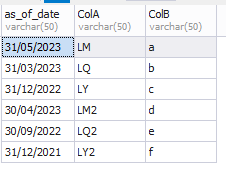I would like help coding a query that filter on the table using the current_date() and retrieve only those rows using the field as_of_date to retrieve end of last quarter i.e.Last Quarter End For example the output would look like the following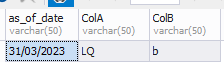I think the solution needs a CASE STATEMENT, but I'm not sure.

Below is the sample table

``````CREATE TABLE #tmpTable (
as_of_date varchar(50),
ColA varchar(50),
ColB varchar(50))

INSERT #tmpTable VALUES
('31/05/2023','LM','a'),
('31/03/2023','LQ','b'),
('31/12/2022','LY','c'),
('30/04/2023','LM2','d'),
('30/09/2022','LQ2','e'),
('31/12/2021','LY2','f')

SELECT * FROM #tmpTable
``````

Any help greatly appreciated.

I would solve it like this:

``````SELECT
YEAR(CONVERT(DATETIME,GETDATE())) * 10 + DATENAME(QUARTER,GETDATE()) AS YEARQUATER_current_date,
YEAR(CONVERT(DATETIME,as_of_date,103)) * 10 + DATENAME(QUARTER,CONVERT(DATETIME,as_of_date,103)) AS YEARQUATER_as_of_date,
*
FROM #tmpTable
WHERE YEAR(CONVERT(DATETIME,as_of_date,103)) * 10 + DATENAME(QUARTER,CONVERT(DATETIME,as_of_date,103)) < YEAR(CONVERT(DATETIME,GETDATE())) * 10 + DATENAME(QUARTER,GETDATE())
``````

Hi RogiePronk,

Thanks for reaching out.

I tried the following code, but it didn't get the results as expected.

I thought I should only get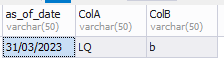This:

`DATEADD(QUARTER, DATEDIFF(QUARTER, 0, CAST(GETDATE() AS date)), 0)`

is the "best practice" / "standard" way to get the first day of the current quarter. Then just subtract one day to get the last day of the previous quarter.

Well, I edited my original post, before the one above, but I guess it just went away instead (??).

``````
SELECT *
FROM #tmpTable
WHERE as_of_date = CONVERT(varchar(10), DATEADD(DAY, -1,
DATEADD(QUARTER, DATEDIFF(QUARTER, 0, CAST(GETDATE() AS date)), 0)), 103)
``````

Hi ScottPletcher,

That worked perfectly thank you.

Can I be cheeky and ask for a related question.
I need another code, based on what you have just done, but modified to retrieve the end of the last quarter but one. The result should look like the following from the sample table: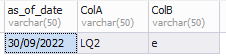So, basically the answer needs to look to year before now (2023),to 2022 and go back one quarter, September.

Does that make sense?

This will give you the start of the quarter for the prior year:

If you need to back that up a quarter or two, then do this:

DATEADD(QUARTER, <code_from_above>, -2 /* or -1 */)

And, as always, to back up one day from that, enclose it all in DATEADD(DAY, -1, ...) and the CONVERT if you need to compare the value to a char column.

Hi ScottPletcher,

All I'm seeing is

Hi Scott

I tried the following, but it didn't work

SELECT *
FROM #tmpTable
WHERE as_of_date = CONVERT(varchar(10), DATEADD(DAY, -1,

Here is another option:

``````DECLARE @today date = getdate();

SELECT @today
, quarter = (month(@today) - 1) / 3 + 1
, quarter_end = dateadd(DAY, -1, dateadd(MONTH, 3, datefromparts(year(@today), (month(@today) - 1) / 3 + 1, 1)))
``````

To get last year:

``````DECLARE @today date = dateadd(YEAR, -1, getdate());

SELECT @today
, quarter = (month(@today) - 1) / 3 + 1
, quarter_end = dateadd(DAY, -1, dateadd(MONTH, 3, datefromparts(year(@today), (month(@today) - 1) / 3 + 1, 1)))
``````

The real problem is that your table doesn't have a date - it has a string that looks like a date. Trying to compare a date to a string will only work if all of the strings are formatted the same and can be implicitly converted to a date.

The format DD/MM/YYYY cannot be implicitly converted to a date - so that leaves you with converting the date to an appropriate string. Or - making sure you store the data in your temp table as an appropriate date (preferred).

To do the convert you need to use style 103:
convert(char(10), {some date}, 103)
convert(date, {some string date}, 103)

To make this easier - make sure your table is storing the data as an appropriate date data type instead of a string.

``````
SELECT *
FROM #tmpTable
WHERE as_of_date = CONVERT(varchar(10), DATEADD(DAY, -1,
``````

Ahhhhhhh ..... I didn't realise that sending the sample table with a string is the reason for the conversion. I just sent the sample table with a string because I thought it would easier for forum members to help me.

In our production environment the date field will be format as a date.

Thanks

I'm getting the following error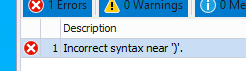Hi ScottPlecher,

Sorry to be a pain, I have provided the sample table, but this time with as_of_date as a date field. Could you please repost you code below without the conversion

``````SELECT *
FROM #tmpTable
WHERE as_of_date = CONVERT(varchar(10), DATEADD(DAY, -1,
DATEADD(QUARTER, DATEDIFF(QUARTER, 0, CAST(GETDATE() AS date)), 0)), 103)
``````
``````CREATE TABLE #tmpTable (
as_of_date date,
ColA varchar(50),
ColB varchar(50))

INSERT #tmpTable VALUES
(CONVERT(DATETIME, '2023-05-31', 120),'LM','A'),
(CONVERT(DATETIME, '2023-03-31', 120),'LQ','B'),
(CONVERT(DATETIME, '2022-12-31', 120),'LY','C'),
(CONVERT(DATETIME, '2023-04-30', 120),'LM2','D'),
(CONVERT(DATETIME, '2022-09-30', 120),'LQ2','E'),
(CONVERT(DATETIME, '2021-12-31', 120),'LY2','F')

SELECT * FROM #tmpTable
``````

Thanks Scott

``````
SELECT *
FROM #tmpTable